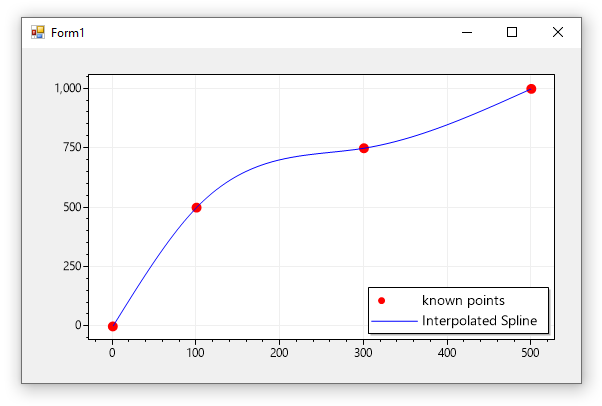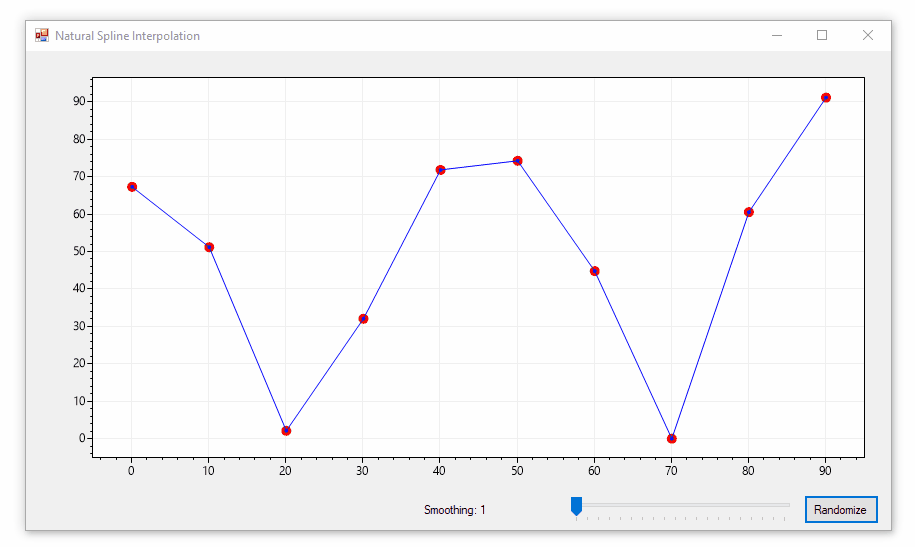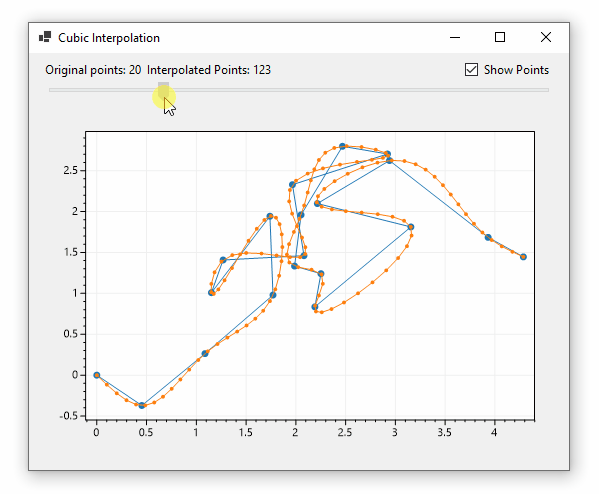{{ message }}

Instantly share code, notes, and snippets.

dreikanter/Interpolator.cs

Last active Jan 22, 2022
Spline interpolation in C#
This file contains bidirectional Unicode text that may be interpreted or compiled differently than what appears below. To review, open the file in an editor that reveals hidden Unicode characters. Learn more about bidirectional Unicode characters
 using System; using System.Collections.Generic; using System.Linq; using System.Text; using System.Diagnostics; namespace Interpolation { /// /// Spline interpolation class. /// public class SplineInterpolator { private readonly double[] _keys; private readonly double[] _values; private readonly double[] _h; private readonly double[] _a; /// /// Class constructor. /// /// Collection of known points for further interpolation. /// Should contain at least two items. public SplineInterpolator(IDictionary nodes) { if (nodes == null) { throw new ArgumentNullException("nodes"); } var n = nodes.Count; if (n < 2) { throw new ArgumentException("At least two point required for interpolation."); } _keys = nodes.Keys.ToArray(); _values = nodes.Values.ToArray(); _a = new double[n]; _h = new double[n]; for (int i = 1; i < n; i++) { _h[i] = _keys[i] - _keys[i - 1]; } if (n > 2) { var sub = new double[n - 1]; var diag = new double[n - 1]; var sup = new double[n - 1]; for (int i = 1; i <= n - 2; i++) { diag[i] = (_h[i] + _h[i + 1]) / 3; sup[i] = _h[i + 1] / 6; sub[i] = _h[i] / 6; _a[i] = (_values[i + 1] - _values[i]) / _h[i + 1] - (_values[i] - _values[i - 1]) / _h[i]; } SolveTridiag(sub, diag, sup, ref _a, n - 2); } } /// /// Gets interpolated value for specified argument. /// /// Argument value for interpolation. Must be within /// the interval bounded by lowest ang highest values. public double GetValue(double key) { int gap = 0; var previous = double.MinValue; // At the end of this iteration, "gap" will contain the index of the interval // between two known values, which contains the unknown z, and "previous" will // contain the biggest z value among the known samples, left of the unknown z for (int i = 0; i < _keys.Length; i++) { if (_keys[i] < key && _keys[i] > previous) { previous = _keys[i]; gap = i + 1; } } var x1 = key - previous; var x2 = _h[gap] - x1; return ((-_a[gap - 1] / 6 * (x2 + _h[gap]) * x1 + _values[gap - 1]) * x2 + (-_a[gap] / 6 * (x1 + _h[gap]) * x2 + _values[gap]) * x1) / _h[gap]; } /// /// Solve linear system with tridiagonal n*n matrix "a" /// using Gaussian elimination without pivoting. /// private static void SolveTridiag(double[] sub, double[] diag, double[] sup, ref double[] b, int n) { int i; for (i = 2; i <= n; i++) { sub[i] = sub[i] / diag[i - 1]; diag[i] = diag[i] - sub[i] * sup[i - 1]; b[i] = b[i] - sub[i] * b[i - 1]; } b[n] = b[n] / diag[n]; for (i = n - 1; i >= 1; i--) { b[i] = (b[i] - sup[i] * b[i + 1]) / diag[i]; } } } }
This file contains bidirectional Unicode text that may be interpreted or compiled differently than what appears below. To review, open the file in an editor that reveals hidden Unicode characters. Learn more about bidirectional Unicode characters
 using System; using System.Collections.Generic; using System.Linq; using System.Text; using System.Diagnostics; namespace Interpolation { class Program { static void Main(string[] args) { var r = 1000; var known = new Dictionary { { 0.0, 0.0 }, { 100.0, 0.50 * r }, { 300.0, 0.75 * r }, { 500.0, 1.00 * r }, }; foreach (var pair in known) { Debug.WriteLine(String.Format("{0:0.000}\t{1:0.000}", pair.Key, pair.Value)); } var scaler = new SplineInterpolator(known); var start = known.First().Key; var end = known.Last().Key; var step = (end - start) / 50; for (var x = start; x <= end; x += step) { var y = scaler.GetValue(x); Debug.WriteLine(String.Format("\t\t{0:0.000}\t{1:0.000}", x, y)); } } } }

Emmie-92 commented Apr 6, 2013

Thnkx ,but m getting an Error type
Error 1 The type or namespace name 'SplineScaler' could not be found (are you missing a using directive or an assembly reference?)
Error The type or namespace name 'EmbeddedCE' could not be found (are you missing a using directive or an assembly reference?)on

dreikanter commented May 25, 2013

Both problems are fixed, sorry for late reply.

JAOT commented Feb 12, 2016

Hello!
When trying your code as-is (thank you for the example, by the way!), I get an error "Index was outside the boundaries of the array". It seems to come from line 94 in Interpolator.cs

ThomasMasuth commented Sep 7, 2016

I get same error at the same point as JAOT wrote.

HughPH commented Jan 1, 2018

If anyone else is experiencing the same issue, note that you can't get an interpolated value for points beyond the bounds of what you've supplied.

I adapted this code to work with an array of PointF. It just happened to be the one I picked up today, there are plenty of other examples online some of which contain protections against you trying the impossible.

Zktchr0 commented Aug 8, 2018

I got the "Index was outside the boundaries of the array" as the others did.

I found how to fix it, and it seems to work more or less fine now, but as I can't say I really understand what's going on there, I'm not sure if I didn't mess up anything.
Anyway - the problem is that in GetValue - if gap is 0 it looks for index -1. I just added on line 92: "if (gap == 0) gap = 1;"...
I suppose it's not that relevant for most of you, but I'd be happy to know if it's a reasonable solution...

Probably there's still something wrong, as I always get infinity for the first element =
But other than that, it seems very nice.

gimulnautti commented Sep 4, 2018

Not being able to interpolate at zero is ok, just add Double.Epsilon to the first key you ask from scaler.GetValue

swharden commented Jun 15, 2020 • edited

I got this working, but I did have to modify the code.

Like @gimulnautti suggested I modified the GetValue() method to add an epsilon if a key of zero was given. This prevented this Gist from crashing, but testing with random data frequently crashed. I then went with @Zktchr0's solution of forcing the gap to be greater than zero before the complicated return statement, but still got crashes. The solution I came up with was:

// these two lines are new
gap = Math.Max(gap, 1);
gap = Math.Min(gap, h.Length - 1);

var x1 = key - previous;
var x2 = h[gap] - x1;

return ((-a[gap - 1] / 6 * (x2 + h[gap]) * x1 + knownYs[gap - 1]) * x2 +
(-a[gap] / 6 * (x1 + h[gap]) * x2 + knownYs[gap]) * x1) / h[gap];

Then I wrote some code to plot the original "known" data points and the interpolated spline. I noticed that some of the values returned by the interpolator are near-infinity, so I added some extra logic to exclude those values. This example plots data with ScottPlot, with a FormsPlot simply drag/dropped into a Form. The code in this Gist doesn't instill me with the highest confidence in the world (after all it does crash immediately), but in a pinch this modification meets my needs.public Form1()
{
InitializeComponent();

var knownPoints = new Dictionary<double, double>
{
{ 0, 0 },
{ 100, 500  },
{ 300, 750  },
{ 500, 1000  },
};

formsPlot1.plt.PlotScatter(
xs: knownPoints.Keys.ToArray(),
ys: knownPoints.Values.ToArray(),
color: Color.Red,
lineWidth: 0,
markerSize: 10,
label: "known points");

var interpolator = new SplineInterpolator(knownPoints);

double deltaX = .5;
List<double> xs = new List<double>();
List<double> ys = new List<double>();
for (double x = knownPoints.Keys.Min(); x < knownPoints.Keys.Max(); x += deltaX)
{
double y = interpolator.GetValue(x);
if (y > -1e10 && y < 1e10) // exclude crazy values
{
}
}

formsPlot1.plt.PlotScatter(
xs: xs.ToArray(),
ys: ys.ToArray(),
color: Color.Blue,
markerSize: 0,
label: "Interpolated Spline");

formsPlot1.plt.Legend();
formsPlot1.Render();
}

crener commented Jun 21, 2020

@swharden I'm looking for an easy/existing of a cubic spline implementation that I can take apart and reuse. So far the best I've found is this https://www.codeproject.com/Articles/560163/Csharp-Cubic-Spline-Interpolation which still isn't great as it's written weirdly but at least all the math is done and works.

swharden commented Jun 22, 2020 • edited

@swharden I'm looking for an easy/existing of a cubic spline implementation that I can take apart and reuse. So far the best I've found is this https://www.codeproject.com/Articles/560163/Csharp-Cubic-Spline-Interpolation which still isn't great as it's written weirdly but at least all the math is done and works.

Hey @crener, since I last wrote that I found one and implemented it in C# as a portable little module. Check out the notes and resources here ScottPlot/ScottPlot#459and I use it like this:

double[] xs = { 0, 10, 20, 30 };
double[] ys = { 65, 25, 55, 80 };

var nsi = new ScottPlot.Statistics.Interpolation.NaturalSpline(xs, ys, resolution: 20);
var psi = new ScottPlot.Statistics.Interpolation.PeriodicSpline(xs, ys, resolution: 20);
var esi = new ScottPlot.Statistics.Interpolation.EndSlopeSpline(xs, ys, resolution: 20);

var plt = new ScottPlot.Plot();
plt.PlotScatter(xs, ys, Color.Black, markerSize: 10, lineWidth: 0, label: "Original Data");
plt.PlotScatter(nsi.interpolatedXs, nsi.interpolatedYs, Color.Red, markerSize: 3, label: "Natural Spline");
plt.PlotScatter(psi.interpolatedXs, psi.interpolatedYs, Color.Green, markerSize: 3, label: "Periodic Spline");
plt.PlotScatter(esi.interpolatedXs, esi.interpolatedYs, Color.Blue, markerSize: 3, label: "End Slope Spline");
plt.Legend();

crener commented Jun 22, 2020 • edited

Oh wow, http://www.mosismath.com/NaturalSplines/NaturalSplines.html Really is a way better example (plus the added example of being in the goto graphing library for c#). I hope that that people looking at this will go over there instead (or the search engines put that result a little higher) :)

Thanks

iliachry commented Aug 30, 2020 • edited

@swharden Thanks for the work on that. I am trying to use your package in Unity3D but the game crashes when I try to run

var psi = new ScottPlot.Statistics.Interpolation.PeriodicSpline(xs, ys, resolution: 20);

I downloaded your package through Nuget and put the .dll with its' dependency at my Unity3D Plugins folder. I also use the ScottPlot.Statistics.Interpolation namespace in the script I want to perform the interpolation.

Do you know if I am doing something wrong or is there something I do not understand?

crener commented Aug 30, 2020

@iliachry Ha, ironically thats what I did, ended up making this https://github.com/crener/Dots-Spline though I did basically refactor the code a lot to integrate it into the existing spline system I had :)

swharden commented Aug 30, 2020

@iliachry I understand you are trying to use ScottPlot's Statistics.Interpolation module in Unity. You said "the game crashes", but that's not really enough information to facilitate a helpful response. Is an error message displayed? Does ScottPlot throw an exception, or is the problem elsewhere in your code?

If you're not sure how to use general NuGet packages in Unity, that topic is probably outside the scope of spline interpolation and this gist. @crener's Dots-Spline package for Unity may be a simpler starting point.

On the other hand if you found a list of X and Y pairs that causes ScottPlot's spine interpolation method to fail, copy/paste those values here or post those values in an issue on ScottPlot's GitHub and I'd be happy to take a look!

swharden commented Aug 30, 2020

@iliachry one more thought is that if your xs or ys arrays contain double.NaN, double.PositiveInfinity, or double.NegativeInfinity, it might explain an exception thrown during interpolation...

A closer look at what values you're sending into Statistics.Interpolation.PeriodicSpline method may be helpful 👍

iliachry commented Aug 31, 2020

@crener thanks a lot. I'll give it a try if I won't make it with ScottPlott.

@swharden finally I found out that Unity crashes because I wanted to Interpolate 10^4 samples. I just now use 5 samples and Unity doesn't crash. Regarding your questions, Unity doesn't throw any exception or displays an error message. However, I don't get a smooth signal yet. I will search a little bit more what's happening and I will reply here if it doesn't finally work. Thanks a lot for your quick response.

swharden commented Aug 31, 2020 • edited

@iliachry thanks for following up! I hadn't tested my implementation against a large number of samples like that. I suspect ScottPlot's spline interpolation module isn't optimized for large datasets, so your math problem took so long to solve that it gave the appearance of "Unity crashing". Good luck on your project! 👍

iliachry commented Aug 31, 2020

@swharden the problem was that Unity didn't write in the console something I wanted, which was in the same script before the creation of the spline. Anyway, now it works very well. Thank you very much for the implementation.

swharden commented Jan 22, 2022

I recently found a more reliable method for achieving cubic spline interpolation in C#. The code I'm linking to here is simpler, faster, stateless, and doesn't throw exceptions.

https://swharden.com/blog/2022-01-22-spline-interpolation